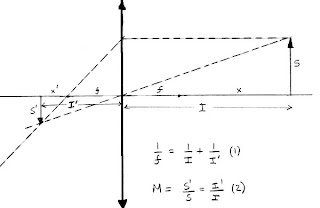## Saturday, February 9, 2008

### Macro Photography with ExtensionWhen an object is at infinity, the image of this object is formed at a distance equal to the focal length (f) behind the lens. As the camera is focused close and closer, the image is formed further and further behind, at a distance x' from f.

Most camera lenses are designed to operate satisfactorily with an object lying between infinity and about 10 focal lengths. The maximum lens focusing distance is then about 1/10 of the focal length, representing a maximum magnification of 0.1. One exception are macro lenses who are designed to work at higher magnifications, usually 0.5 or 1.0.

To make a lens focus closer than its minimum focusing distance, one can use extension tubes or bellows. This is of course possible only if the lens can be removed from the camera. The basic equations in work here are the fundamental lens formula and the definition of magnification. I will write these as follows (see also this blog):

1/f = 1/I + 1/I'
and:
M = s'/s = I'/I = f/x = x'/f

I am using this notation: f = focal length, x' = lens extension (distance of lens from film plane), I = Subject distance (distance of subject from lens), also known as working distance because it shows you how much room to you have to work with your subject, M is the magnification.

An interesting situation exists at M=1. In this case, I = I' = 2F, i.e. the extension is equal to the working distance and it is equal to two times the focal length. For example, if you are using a 50mm macro lens at M=1, then the subject is at 100mm in front of the lens and the image is formed 100mm behind the lens. As it turns out, the total distance from object to film plane (4F or 200mm) is the smallest possible. As you increase the magnification beyond 1, the image moves quickly behind the lens and a bellows system will be needed. For best optical performance, it is recommended that the lens is reversed when M > 1

Another note of practical importance: At magnifications close to 1 or higher, focusing is not done by changing the distance of the lens to the film plane, because this changes the magnification. Focusing is done but my moving the lens and camera closer to the object. So, in macro stereo photography, first you set the magnification by fixing the extension I', and then you focus by changing the working distance I. For stereo photography with a slide bar, you might need a stereo bar with two axes. The vertical axis is used for stereo translation. The horizontal axis is used for focusing.